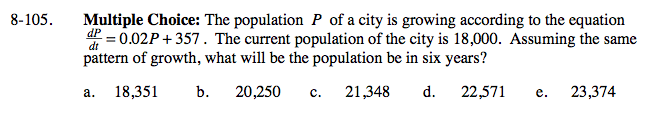### Home > CALC > Chapter 8 > Lesson 8.3.1 > Problem8-105

8-105.Notice that the given equation is a derivative. It gives information about the rate of change of the population. But what we want to know is information about the actual population. How do we undo a derivative?

It is necessary to use implicit integration because the derivative is in terms of time, but time does not appear in the equation.

$\frac{dP}{dt}=0.02P+357$

$\frac{dP}{dt}=0.02(P+17850)$

$\text{Separate the sides: }\frac{1}{P+17850}dP=0.02dt$

Integrate both sides.

Combine the constants of integration.

Evaluate the given point to solve for C: C = 35800

Substitute in the value of C, then write an equation for P(t).

Use your P(t) equation to find Population at t = 6 years.